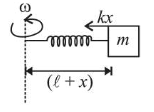# A particle of mass m is fixed to one end of a light spring having force constant k and unstretched length l.Question:

A particle of mass $m$ is fixed to one end of a light spring having force constant $k$ and unstretched length $l$. The other end is fixed. The system is given an angular speed $\omega$ about the fixed end of the spring such that it rotates in a circle in gravity free space. Then the stretch in the spring is:

1. $\frac{m l \omega^{2}}{k-\omega m}$

2. $\frac{m l \omega^{2}}{k-m \omega^{2}}$

3. $\frac{m l \omega^{2}}{k+m \omega^{2}}$

4. $\frac{m l \omega^{2}}{k+m \omega}$

Correct Option: , 2

Solution:

(2) At elongated position $(x)$,

$F_{\text {radial }}=\frac{m v^{2}}{r}=m r \omega^{2}$$\therefore k x=m(\ell+x) \omega^{2}$

$(\because r=\ell+x$ here $)$

$\mathrm{kx}=\mathrm{m} \ell \omega^{2}+\mathrm{mx} \omega^{2}$

$\therefore x=\frac{m \ell \omega^{2}}{k-m \omega^{2}}$